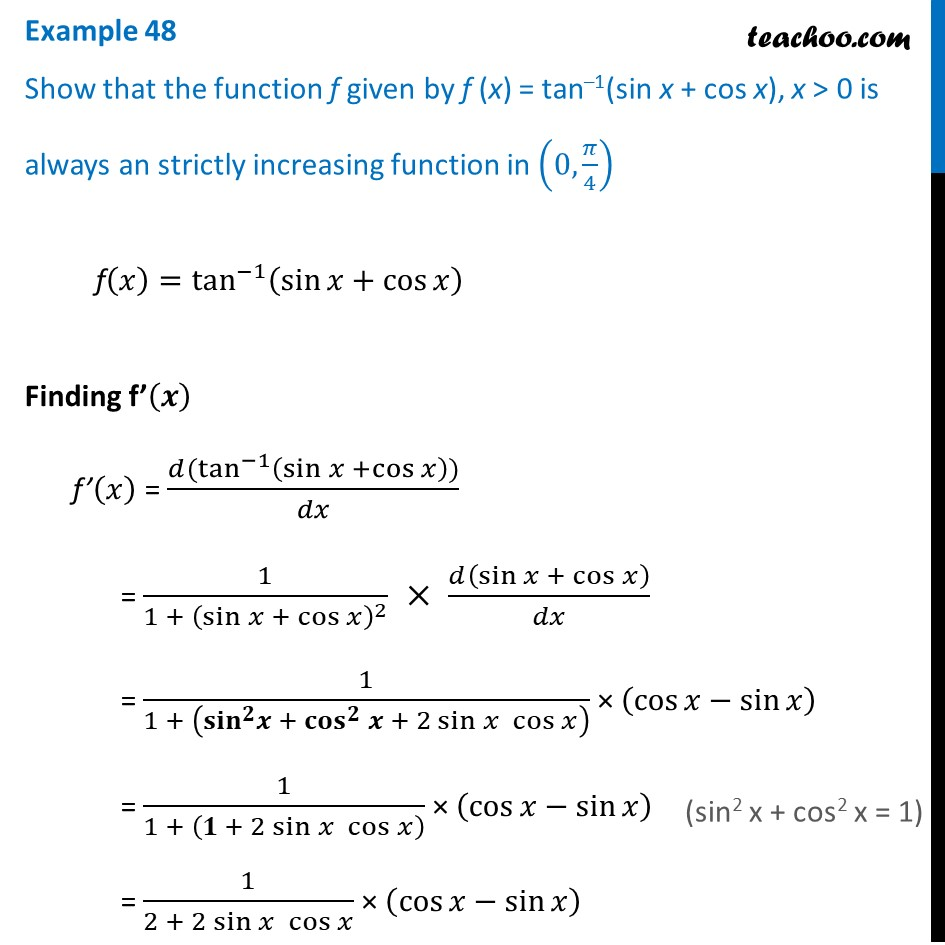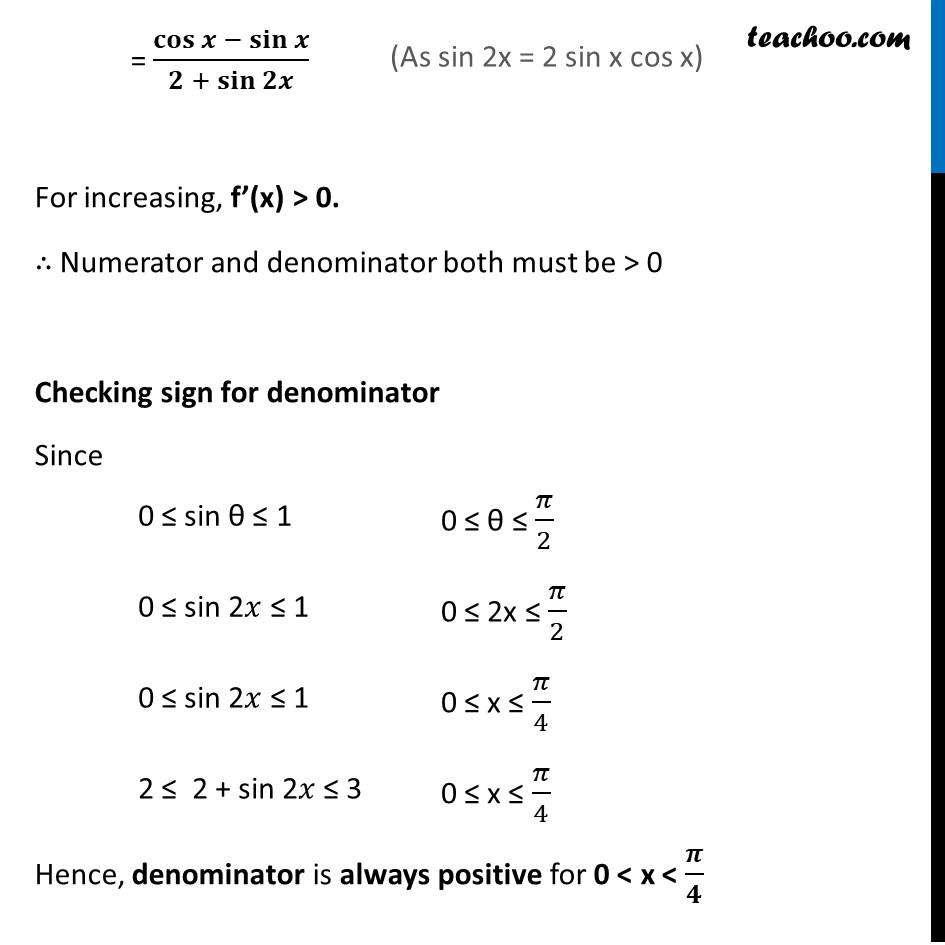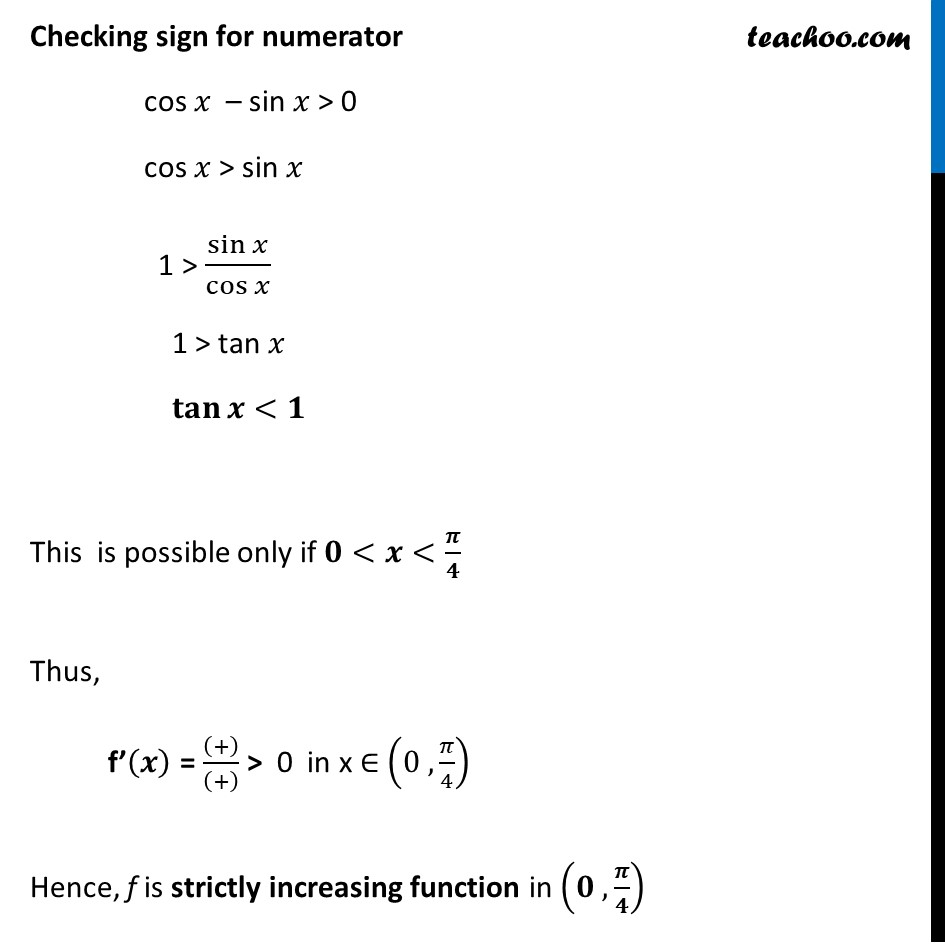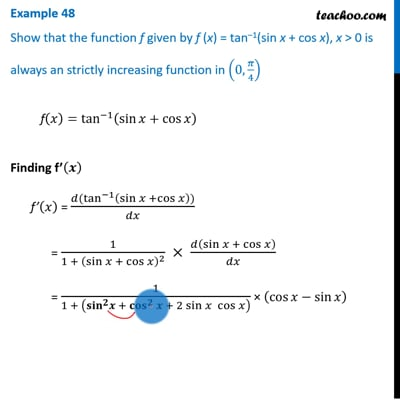Examples

Chapter 6 Class 12 Application of Derivatives
Serial order wiseThis video is only available for Teachoo black users

Maths Crash Course - Live lectures + all videos + Real time Doubt solving!

### Transcript

Example 48 Show that the function f given by f (x) = tan–1(sin x + cos x), x > 0 is always an strictly increasing function in (0,𝜋/4) f(𝑥)=tan^(−1)⁡(sin⁡𝑥+cos⁡𝑥 ) Finding f’(𝒙) f’(𝑥) = (𝑑(tan^(−1)⁡〖(sin⁡𝑥 +cos⁡𝑥 ))〗)/𝑑𝑥 = 1/(1 + (sin⁡〖𝑥 + cos⁡𝑥 〗 )^2 ) × 𝑑(sin⁡〖𝑥 + cos⁡𝑥 〗 )/𝑑𝑥 = 1/(1 + (〖〖𝐬𝐢𝐧〗^𝟐 𝒙〗⁡〖+〖𝐜𝐨𝐬〗^𝟐⁡〖𝒙 〗+ 2 sin⁡𝑥 cos⁡𝑥 〗 ) ) × (cos⁡𝑥−sin⁡𝑥 ) = 1/(1 + (𝟏 + 2 sin⁡𝑥 cos⁡𝑥 ) ) × (cos⁡𝑥−sin⁡𝑥 ) = 1/(2 + 2 sin⁡𝑥 cos⁡𝑥 ) × (cos⁡𝑥−sin⁡𝑥 ) = 𝐜𝐨𝐬⁡〖𝒙 −〖 𝐬𝐢𝐧〗⁡𝒙 〗/(𝟐 + 𝐬𝐢𝐧⁡𝟐𝒙 ) For increasing, f’(x) > 0. ∴ Numerator and denominator both must be > 0 Checking sign for denominator Since Hence, denominator is always positive for 0 < x < 𝝅/𝟒 Checking sign for numerator cos 𝑥 – sin 𝑥 > 0 cos 𝑥 > sin 𝑥 1 > sin⁡𝑥/cos⁡𝑥 1 > tan 𝑥 𝐭𝐚𝐧⁡𝒙<𝟏 This is possible only if 𝟎<𝒙<𝝅/𝟒 Thus, f’(𝒙) = ((+))/((+) ) > 0 in x ∈ (0 , 𝜋/4) Hence, f is strictly increasing function in (𝟎 , 𝝅/𝟒)